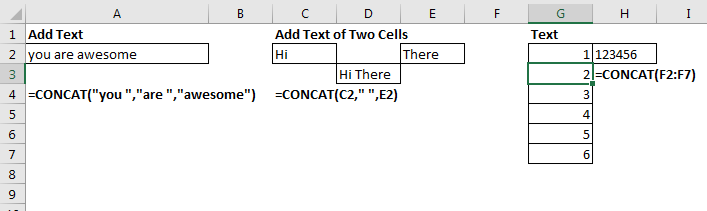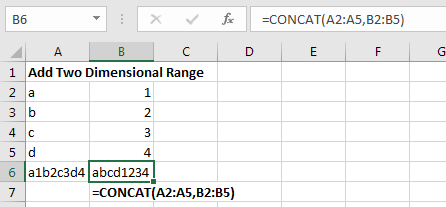# The Excel CONCAT Function

The new Excel CONCAT function is introduced in Excel 2019 and Excel 365. The CONCAT function simply adds given strings into one, just like CONCATENATE function but better. Unlike CONCATENATE function, the CONCAT function can a range as argument and return a joined text of each cell into one.

Syntax of the CONCAT function

=CONCAT(text1,[text2],...)

Text1 : The text1 can be any text or range that you want to concatenate.
[text2]: This is optional. This also can be any text or range.

You can add as many as ranges using commas.

Let’s understand this function with some examples.
Examples of the CONCAT functionExample 1: Simply add two or more texts:

=CONCAT("you ","are ","awesome")

The formula will return the sentence "You are awesome" (which you certainly are).
Example 2: Add Text From Two or More Different Cells:

If you want to add text from two or more different cells that do not touch each other then simply give reference of those cells with commas in between.

Here I have "Hi" in cell C2 and "There" in cell E2. I want to add them using the CONCAT function. The formula will be:

=CONCAT(C2," ",E2)

Here, I have used " " as a separator so that we get output as "Hi There" not "HiThere".
Now all this can be done using CONCATENATE function too. How is CONCAT different? Next example tells how.

Example 3: Add texts of a Range
Here I have a series of numbers in range F2:F7. I want these numbers to be joined as 123456. So I simply give the range reference to the CONCAT function.

=CONCAT(F2:F7)

This was not possible with CONCATENATE function.

Example 3: Add Text of Two Dimensional Range Horizontally.

Let's say you a,b,c,d in range A2:A5 and 1,2,3,4 in range B2:B5 and you want to concatenate these two ranges so that you get output as a1b2c3d4. In that case we simply give the entire range as reference to the CONCAT function.

=CONCAT(A2:B5)

This will return the output a1b2c3d4.

Example 4: Add Text of Two Dimensional Range Vertically.

In the above example, if you wanted to add these texts vertically so that you get output as "abcd1234". In that case, you will need to give the reference of these two ranges separately.

=CONCAT(A2:A5,B2:B5)

This will return the output as "abcd1234"Note: This function is available in Excel 2019 and 365.

So yeah guys this how we use the CONCAT function in Excel. I hope it was helpful. If you have any doubts regarding this article or have any Excel/VBA query, ask in the comments section below.

Excel 2019/365 Functions:

How to Use The Excel UNIQUE Function | The UNIQUE function returns the Unique values from the given array.

The SORT Function in Excel | The SORT function returns the sorted form of the supplied array.

How to Use Excel SORTBY Function | The SORTBY function sorts a given range by a different specified range. Sorting range does not need to be part of the table.

How to Use The Excel FILTER Function | The FILTER function returns all matched values of given criteria and spills the result into adjacent cells.

The SEQUENCE Function in Excel | The SEQUENCE function returns a series of sequential numbers. It is a dynamic array formula.

Popular Articles:

50 Excel Shortcuts to Increase Your Productivity | Get faster at your task. These 50 shortcuts will make you work even faster on Excel.

How to use the VLOOKUP Function in Excel | This is one of the most used and popular functions of excel that is used to lookup value from different ranges and sheets.

How to use the COUNTIF function in Excel 2016 | Count values with conditions using this amazing function. You don't need to filter your data to count specific values. Countif function is essential to prepare your dashboard.

How to use the SUMIF Function in Excel | This is another dashboard essential function. This helps you sum up values on specific conditions.

Terms and Conditions of use

The applications/code on this site are distributed as is and without warranties or liability. In no event shall the owner of the copyrights, or the authors of the applications/code be liable for any loss of profit, any problems or any damage resulting from the use or evaluation of the applications/code.